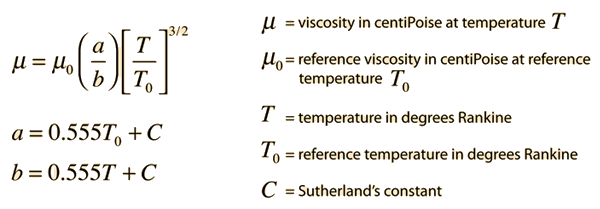# Viscosity of Gases

The viscosity of a gas can be thought of as a measure of its resistance to flow and is measured in the CGS unit Poise = dyne sec/cm2. The viscosity of gases near room temperature are in the centiPoise range, so that is a commonly used unit. Gas viscosity is only weakly dependent on pressure near atmospheric pressure. It is primarily a function of temperature, and can be modeled in terms of temperature with the input of experimental reference measurements. Note that as an engineering quantity, the temperatures used are in the Rankine scale.

Gas viscosity can be modeled by Sutherland's formula:Since Sutherland's formula is an empirical fit of measured data, the following table of reference data is needed.

 Gas Sutherland'sconstant C T0°R μ0centiPoise standard air 120 524.07 0.01827 ammonia, NH3 370 527.67 0.00982 carbon dioxide, CO2 240 527.67 0.01480 carbon monoxide, CO 118 518.67 0.01720 hydrogen, H2 72 528.93 0.00876 nitrogen, N2 111 540.99 0.01781 oxygen, O2 127 526.05 0.02018 sulfur dioxide, SO2 416 528.57 0.01254

For temperature T=K = C =F =R ,

a gas with reference viscosity μ0 = centiPoise,

reference temperature T0 = Rankine,

and Sutherland's constant C =

should have a viscosity μ = centiPoise.

References:

For gas viscosity: Chemical Rubber Company (CRC). 1984. CRC Handbook of Chemistry and Physics. Weast, Robert C., editor. 65th edition. CRC Press, Inc. Boca Raton, Florida. USA.

For Sutherland's formula and values for : Crane Company. 1988. Flow of fluids through valves, fittings, and pipe. Technical Paper No. 410 (TP 410)

 Mean free path related to viscosity
Index

Gas law concepts

Kinetic theory concepts

 HyperPhysics***** Thermodynamics R Nave
Go Back